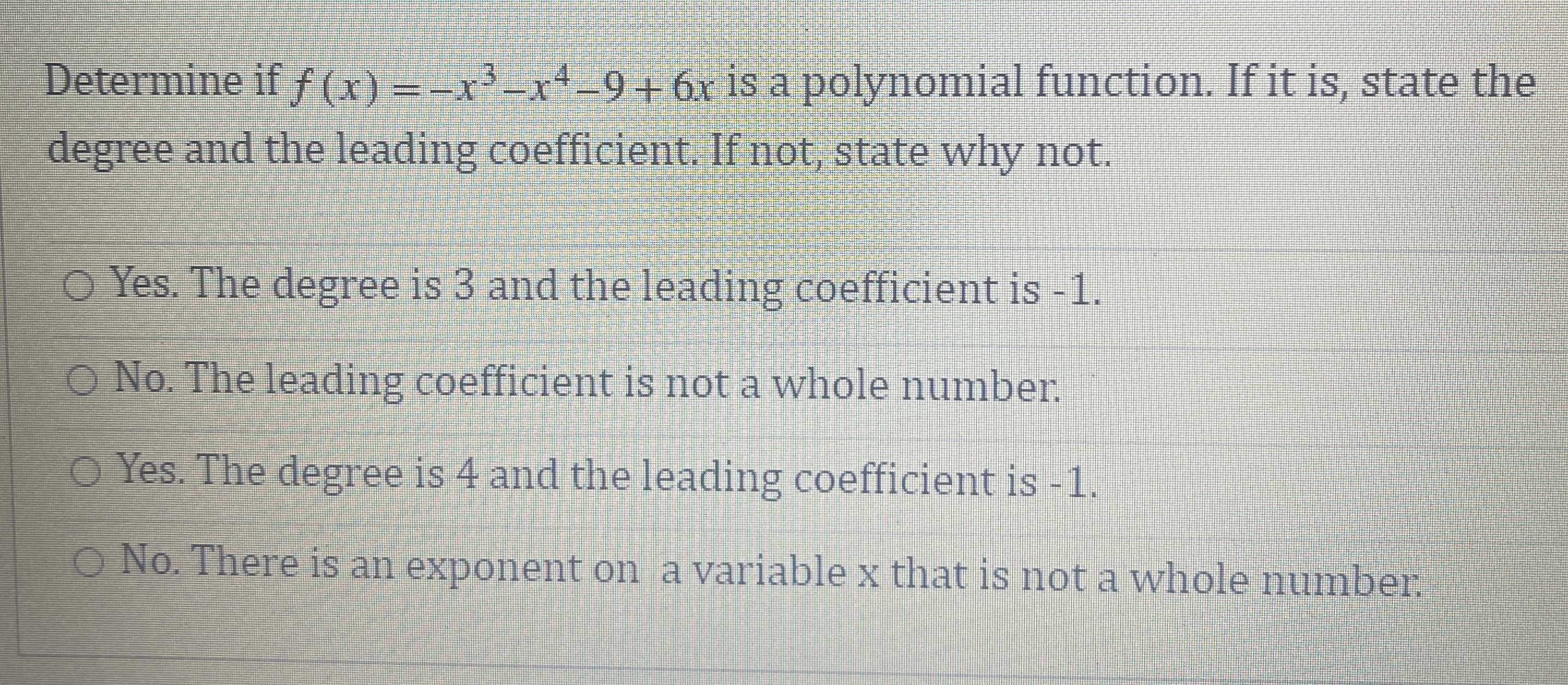### Still have math questions?

Algebra
QuestionDetermine if $$f ( x ) = - x ^ { 3 } - x ^ { 4 } - 9 + 6 x$$ is a polynomial function. If it is, state the degree and the leading coefficient. If not, state why not.

Yes. The degree is $$3$$ and the leading coefficient is $$- 1$$ .

No. The leading coefficient is not a whole number.

Yes. The degree is $$4$$ and the leading coefficient is $$- 1$$ .

No. There is an exponent on a variable $$x$$ that is not a whole number.

$$\\ \text { Yes. The degree is } 4 \text { and the leading coefficient is } - 1$$